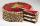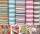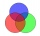# Fraction calculator

The calculator performs basic and advanced operations with fractions, expressions with fractions combined with integers, decimals, and mixed numbers. It also shows detailed step-by-step information about the fraction calculation procedure. Solve problems with two, three, or more fractions and numbers in one expression.

## Result:

### 42/3 - 14/5 = 43/15 = 2 13/15 ≅ 2.8666667

Spelled result in words is forty-three fifteenths (or two and thirteen fifteenths).

### How do you solve fractions step by step?

1. Conversion a mixed number 4 2/3 to a improper fraction: 4 2/3 = 4 2/3 = 4 · 3 + 2/3 = 12 + 2/3 = 14/3

To find new numerator:
a) Multiply the whole number 4 by the denominator 3. Whole number 4 equally 4 * 3/3 = 12/3
b) Add the answer from previous step 12 to the numerator 2. New numerator is 12 + 2 = 14
c) Write a previous answer (new numerator 14) over the denominator 3.

Four and two thirds is fourteen thirds
2. Conversion a mixed number 1 4/5 to a improper fraction: 1 4/5 = 1 4/5 = 1 · 5 + 4/5 = 5 + 4/5 = 9/5

To find new numerator:
a) Multiply the whole number 1 by the denominator 5. Whole number 1 equally 1 * 5/5 = 5/5
b) Add the answer from previous step 5 to the numerator 4. New numerator is 5 + 4 = 9
c) Write a previous answer (new numerator 9) over the denominator 5.

One and four fifths is nine fifths
3. Subtract: 14/3 - 9/5 = 14 · 5/3 · 5 - 9 · 3/5 · 3 = 70/15 - 27/15 = 70 - 27/15 = 43/15
For adding, subtracting, and comparing fractions, it is suitable to adjust both fractions to a common (equal, identical) denominator. The common denominator you can calculate as the least common multiple of both denominators - LCM(3, 5) = 15. In practice, it is enough to find the common denominator (not necessarily the lowest) by multiplying the denominators: 3 × 5 = 15. In the next intermediate step, the fraction result cannot be further simplified by canceling.
In words - fourteen thirds minus nine fifths = forty-three fifteenths.

#### Rules for expressions with fractions:

Fractions - use the slash “/” between the numerator and denominator, i.e., for five-hundredths, enter 5/100. If you are using mixed numbers, be sure to leave a single space between the whole and fraction part.
The slash separates the numerator (number above a fraction line) and denominator (number below).

Mixed numerals (mixed fractions or mixed numbers) write as non-zero integer separated by one space and fraction i.e., 1 2/3 (having the same sign). An example of a negative mixed fraction: -5 1/2.
Because slash is both signs for fraction line and division, we recommended use colon (:) as the operator of division fractions i.e., 1/2 : 3.

Decimals (decimal numbers) enter with a decimal point . and they are automatically converted to fractions - i.e. 1.45.

The colon : and slash / is the symbol of division. Can be used to divide mixed numbers 1 2/3 : 4 3/8 or can be used for write complex fractions i.e. 1/2 : 1/3.
An asterisk * or × is the symbol for multiplication.
Plus + is addition, minus sign - is subtraction and ()[] is mathematical parentheses.
The exponentiation/power symbol is ^ - for example: (7/8-4/5)^2 = (7/8-4/5)2

#### Examples:

subtracting fractions: 2/3 - 1/2
multiplying fractions: 7/8 * 3/9
dividing Fractions: 1/2 : 3/4
exponentiation of fraction: 3/5^3
fractional exponents: 16 ^ 1/2
adding fractions and mixed numbers: 8/5 + 6 2/7
dividing integer and fraction: 5 ÷ 1/2
complex fractions: 5/8 : 2 2/3
decimal to fraction: 0.625
Fraction to Decimal: 1/4
Fraction to Percent: 1/8 %
comparing fractions: 1/4 2/3
multiplying a fraction by a whole number: 6 * 3/4
square root of a fraction: sqrt(1/16)
reducing or simplifying the fraction (simplification) - dividing the numerator and denominator of a fraction by the same non-zero number - equivalent fraction: 4/22
expression with brackets: 1/3 * (1/2 - 3 3/8)
compound fraction: 3/4 of 5/7
fractions multiple: 2/3 of 3/5
divide to find the quotient: 3/5 ÷ 2/3

The calculator follows well-known rules for order of operations. The most common mnemonics for remembering this order of operations are:
PEMDAS - Parentheses, Exponents, Multiplication, Division, Addition, Subtraction.
BEDMAS - Brackets, Exponents, Division, Multiplication, Addition, Subtraction
BODMAS - Brackets, Of or Order, Division, Multiplication, Addition, Subtraction.
GEMDAS - Grouping Symbols - brackets (){}, Exponents, Multiplication, Division, Addition, Subtraction.
Be careful, always do multiplication and division before addition and subtraction. Some operators (+ and -) and (* and /) has the same priority and then must evaluate from left to right.

## Fractions in word problems:

• Length subtractingExpress in mm: 5 3/10 cm - 2/5 mmWhat is 4 1/2+2/7-213/14?
• Pizza fractionsAnn ate a third of a pizza and then another quater. Total part of pizza eaten by Ann and how much pizza is left?
• SchoolThere are 150 pupils in grade 5 . 2/3 of them are female. By what fractions are the males?To three-eighths of one third, we add five quarters of one half and multiply the sum by four. How much will we get?
• Difference mixed fractionsWhat is the difference between 4 2/3 and 3 1/6?
• Cake fractionsThomas ate 1/3 of cake, Bohouš of the rest of the cake ate 2/5. What fraction of cake left over for others?
• Pounds3 pounds subtract 1/3 of a pound.
• EmployeesOf all 360 employees, there are 11/12 women. How many men work in a company?
• PackageThe package was 23 meters of textile. The first day sold 12.3 meters. How many meters of textile remained in the package?
• FortyForty five of the 80 students were girls. What is the ratio of girls to boys?
• Find the 24Find the difference between 2/7 and 1/21
• Coloured teacupsThe teacups in Tea Stop 55 are `2/5` green and `3/10` yellow. What fraction of the teacups are neither green nor yellow?